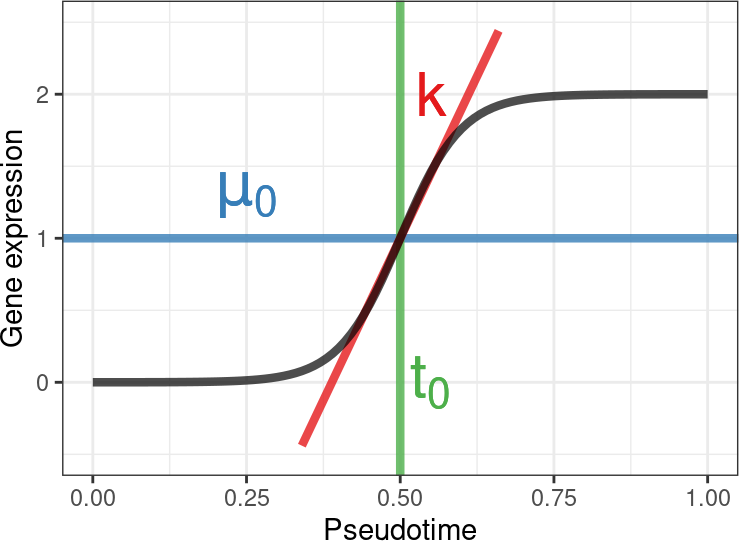# 1 Introduction

switchde is an R package for detecting switch-like differential expression along single-cell RNA-seq trajectories. It assumes genes follow a sigmoidal pattern of gene expression and tests for differential expression using a likelihood ratio test. It also returns maximum likelihood estimates (MLE) for the sigmoid parameters, which allows filtering of genes for up or down regulation as well as where along the trajectory the regulation occurs.

The parametric form of gene expression assumed is a sigmoid:

example_sigmoid()Governed by three parameters:

• $$\mu_0$$ The half-peak expression
• $$k$$ The ‘activation strength’. If positive, the gene is upregulated along the trajectory; if negative, the gene is downregulated. The magnitude of $$k$$ corresponds to how fast the gene is up or down regulated.
• $$t_0$$ The ‘activation time’, or where in the trajectory this behaviour occurs. Note this parameter should be interpreted with respect to the overall range of the pseudotimes supplied.

# 2 Installation

switchde can be installed from both Bioconductor and Github.

Example installation from Bioconductor:

if (!requireNamespace("BiocManager", quietly=TRUE))
install.packages("BiocManager")
BiocManager::install("switchde")

Example installation from Github:

devtools::install_github("kieranrcampbell/switchde")

# 3 Pre-filtering of genes

Several inputs will cause issues in maximum likelihood and expecatation maximisation algorithms typically leading to error messages such as ‘finite gradient required’. To avoid these, strict pre-filtering of genes is advised such as retaining genes with a certain mean expression and expressed in a certain proportion of cells. For example, if the matrix X represents logged expression data, we can retain only genes with mean expression greater than 1 and expressed in 20% of cells via

X_filtered <- X[rowMeans(X) > 0.1 & rowMeans(X > 0) > 0.2,]

By default, switchde also sets any expression less than 0.01 to 0. This can be controlled via the lower_threshold parameter.

# 4 Example usage

We provide a brief example on some synthetic single-cell data bundled with the package. synth_gex contains a 12-by-100 expression matrix of 12 genes, and ex_pseudotime contains a pseudotime vector of length 100. We can start by plotting the expression:

data(synth_gex)
data(ex_pseudotime)

gex_cleaned <- as_data_frame(t(synth_gex)) %>%
dplyr::mutate(Pseudotime = ex_pseudotime) %>%
tidyr::gather(Gene, Expression, -Pseudotime)
## Warning: as_data_frame() was deprecated in tibble 2.0.0.
## Please use as_tibble() instead.
## The signature and semantics have changed, see ?as_tibble.
ggplot(gex_cleaned, aes(x = Pseudotime, y = Expression)) +
facet_wrap(~ Gene) + geom_point(shape = 21, fill = 'grey', color = 'black') +
theme_bw() + stat_smooth(color = 'darkred', se = FALSE)
## geom_smooth() using method = 'loess' and formula 'y ~ x'